## 1. Overview

In this tutorial, we’ll first discuss the paging technique responsible for memory management in the operating system. Then, we’ll present the fragmentation problem and its two variations. Finally, we’ll talk about the core differences between the two variations of fragmentation.

Finally, we’ll explain why only internal fragmentation occurs in paging.

## 2. Introduction to Paging

Paging allows us to store processes in memory in a discontinuous space. To implement this technique, we divide the processes into pages. Pages are blocks of fixed sizes. Similarly, we also divide physical memory into frames.

When executing a process, the CPU converts each page’s logical address to a physical address. Then, using the segmented paging technique, we partition the address provided by the CPU into a page number and a page offset.

If the virtual address is large, pages will take a large space in actual memory in paging. Complex programs tend to be large and take a lot of memory. Hence, managing the main memory with paging is a difficult task for an OS.

Although paging solves the external fragmentation issue, internal fragmentation remains one of the main flaws of paging. Now to understand why there is no external fragmentation in paging, we need to know both of the fragmentations in detail.

To convert a logical address to a reel address, we need to know the page number. We can find the page number associated with a logical address in the page table. We also store the physical address of each page there. Now in order to get the physical address, we extract the corresponding real page addresses and add them to the offset: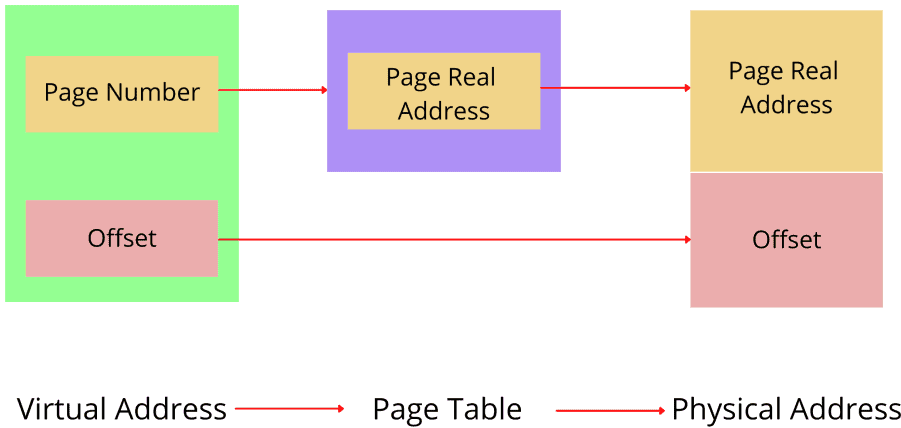### 2.2. Example

Let’s assume we divide a process into three pages: A, B, and C. Each page corresponding to a process has a page number (A, 5), (B, 6), (C, 7) associated with it. Now to find a page in the page table, the CPU uses the page number associated with a page. The segment table stores the page and frame number of each page.

CPU extracts the frame number of each page from the segment table to find them in the physical memory. The offset helps to find the size of the frames, which is already determined: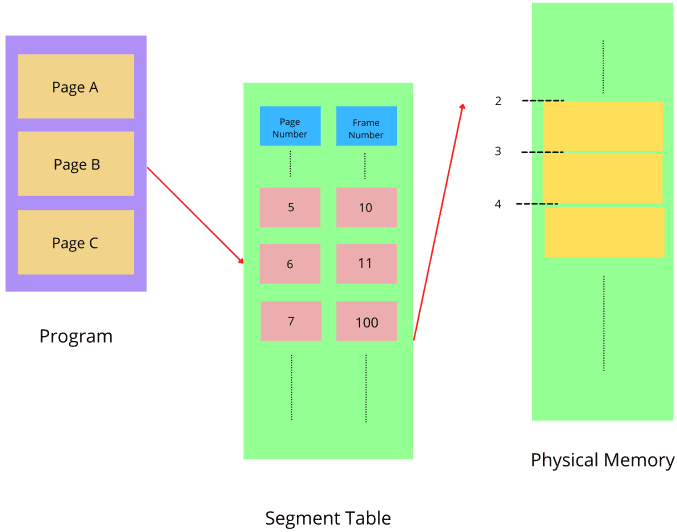## 3. Fragmentation and Its Variations

Fragmentation occurs when we break the memory into small-sized blocks. Furthermore, fragmentations are non-contiguous in nature. Hence, we can’t allocate them to processes. As a result, memory will have multiple unused blocks while processes are pending in the queue and waiting for the memory. Fragmentation can be either internal or external.

Internal fragmentation occurs when we split the physical memory into contiguous mounted-sized blocks and allocate memory for a process that can be larger than the memory requested. Hence, the unused allocated space is left and can’t be used by other processes. Best fit block search is the solution for internal fragmentation.

External fragmentation occurs when total unused memory space is enough to answer all the allocation requests. Here the memory is non-contiguous. Therefore, the memory has empty blocks scattered all over, which are insufficient to be allocated to other programs. Compaction is the solution for external fragmentation.

## 4. Differences Between Internal and External Fragmentation

Let’s talk about the main differences between these two types of fragmentation:## 5. Why Paging Causes Internal Fragmentation?

We already know paging divides programs into fixed-size pages. However, at some point, it would occur that a program wouldn’t need precisely the whole page. Therefore, it can leave a free partition of the memory, leading to memory with allocated unused spaces. This is precisely how internal fragmentation occurs in memory.

Let’s take an example. We’re taking a program that is divided into two blocks of equal size 2KB each. Hence, we allocate 4KB of non-contiguous space in the memory. But if a program needs only 3KB, then an allocated and unused 1KB space is left on memory, causing internal fragmentation: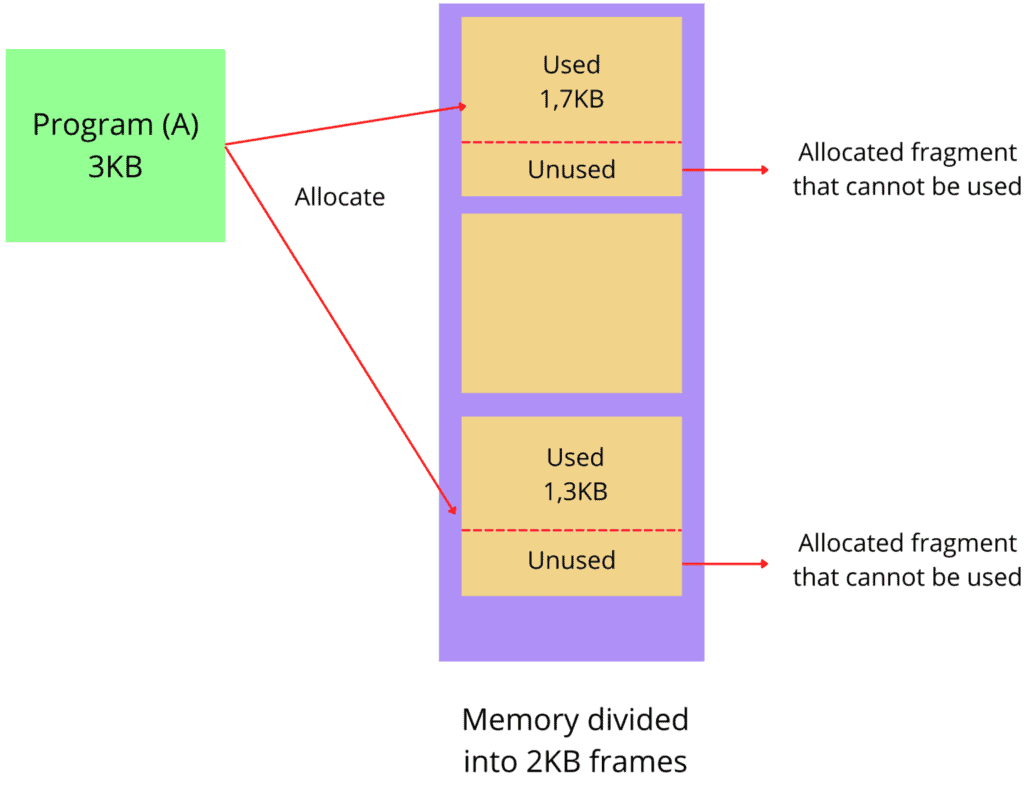Here, there are two blocks of 2KB each. However, the program used 1.7KB from the first block and 1.3KB from the second block. Therefore, there are two allocated but unused small memory chunks left on both blocks. We can’t use these memory chunks to allocate other programs. Hence, the way paging works makes it very vulnerable to internal fragmentation.

## 6. Can External Fragmentation Occur in Paging?

When dividing programs into fixed-size pages, we also divide physical memory into equal size frames. The particularity of this technique is that the space allocated for programs is non-contiguous. Therefore, when other processes don’t allocate non-contiguous blocks, they can be used by other programs. Paging allows them to use non-contiguous memory blocks.

Let’s take an example. First, we divide a program into two non-contiguous fixed-size blocks. Then, any other programs can use the remaining blocks that are not allocated: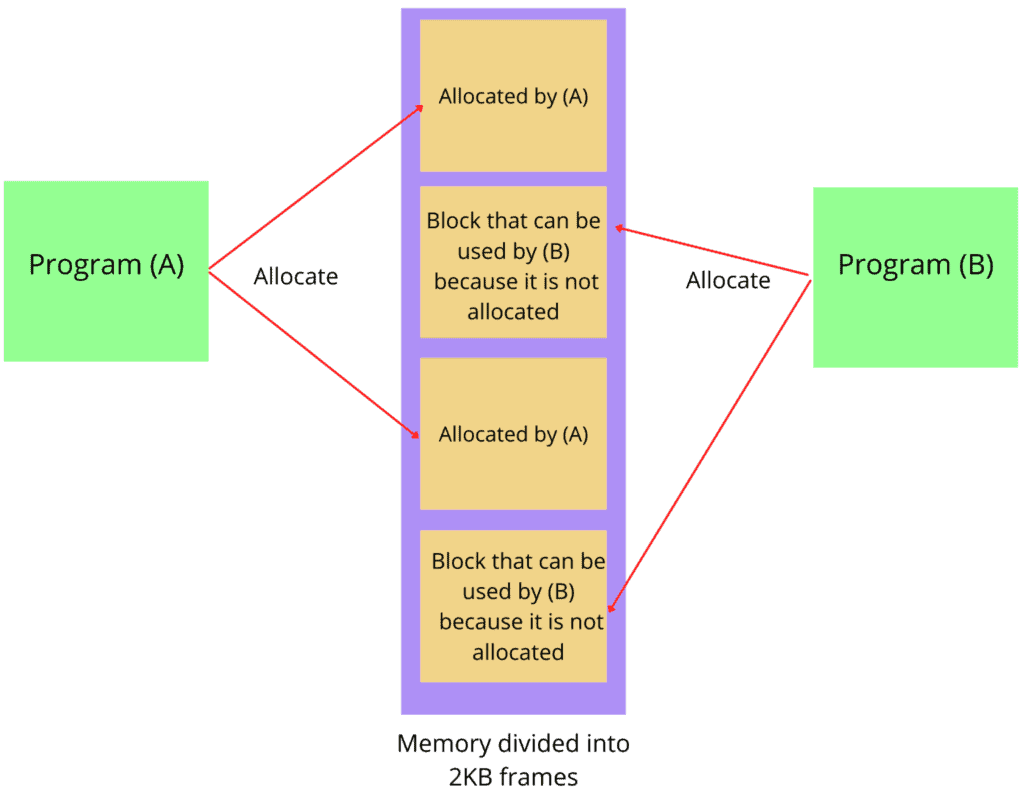Here Program A allocates some space from two non-contiguous fixed-size blocks. However, there is some space left in the blocks. Now because the memory is non-contiguous, Program B can take the remaining space from the blocks. Hence, the probability of external fragmentation in paging is significantly less.

Now let’s merge the external and internal fragmentation examples into one so that we can get a clear idea: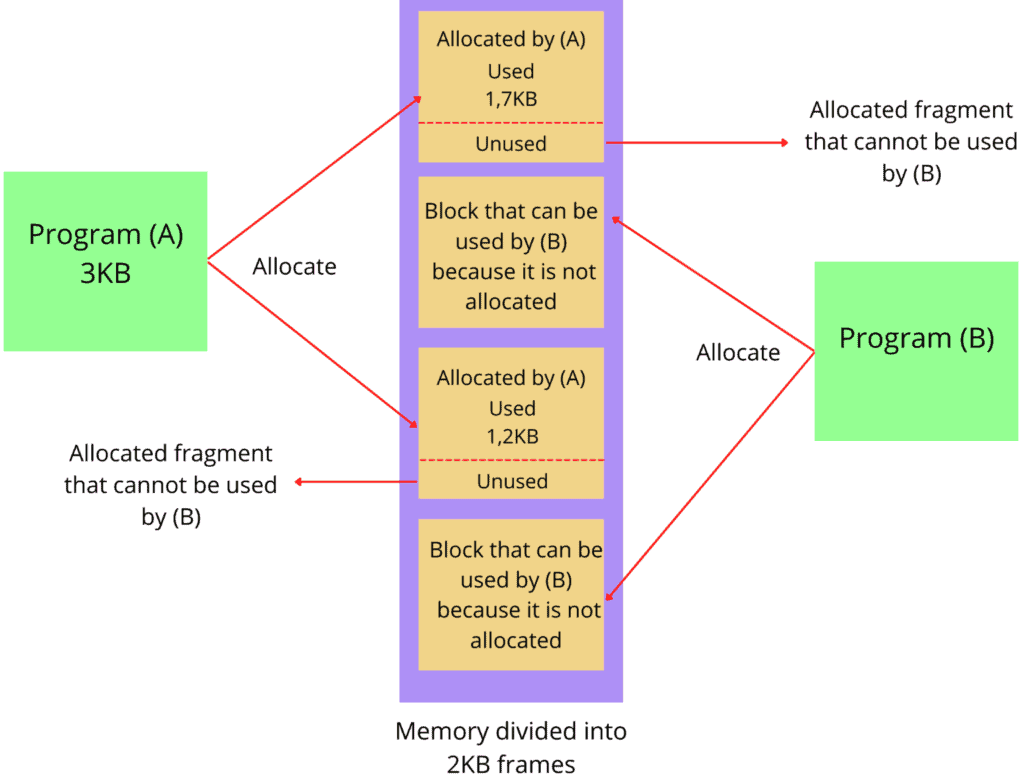## 7. Conclusion

In this tutorial, we discussed fragmentation and its variation: internal and external fragmentation. We also explained why paging is more vulnerable to internal fragmentation.

1 Comment
Inline Feedbacks